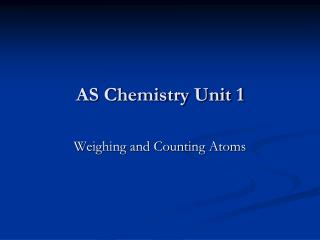DownloadDownload PresentationAS Chemistry Unit 1

# AS Chemistry Unit 1

Télécharger la présentation## AS Chemistry Unit 1

- - - - - - - - - - - - - - - - - - - - - - - - - - - E N D - - - - - - - - - - - - - - - - - - - - - - - - - - -
##### Presentation Transcript

1. AS Chemistry Unit 1 Weighing and Counting Atoms

2. Atoms: The Building Blocks of Matter • Dalton’s Atomic Theory – English school teacher John Dalton was the first to recognize that these laws and many experimental results could be explained by the existence of atoms.

3. Bell Work, 8/15/12 Answer Question # 1 on pg. 21.

4. Statements of the Atomic Theory • All matter is composed of extremely small particle called atoms. • Atoms of a given element are identical in size, mass and other properties; atoms of different elements differ in size, mass, and other properties. • Atoms cannot be subdivided, created or destroyed. • Atoms of different elements can combine in simple, whole-number ratios to form chemical compounds. • In chemical reactions, atoms are combined, separated, or rearranged.

5. Dalton’s Atomic Theory Cont’d • Dalton’s theory has since been discarded it was the basis for further experiments because it successfully explained the Law of Conservation of Mass, Law of Definite Composition and other observations.

6. Theory Updated • We now know that atoms are divisible. • We also know that an element can have atoms with differing masses; these atoms are called isotopes.

7. Theory Laws • Law of Definite Composition by Mass states that a chemical compound contains the same elements in exactly the same proportions by mass regardless of the size of the sample. • Law of Multiple Proportions states that If two or more different compounds are composed of the same two elements, the masses of the second element combined with a certain mass of the first element can be expressed as ratios of small whole numbers.

8. Example: Carbon Monoxide CO Carbon Dioxide CO2 Oxygen O2 Ozone O3

9. Atomic Numbers and Mass Numbers • Atomic Number – (Z) the number of protons in the nucleus of each atom of that element. • Mass Number – total number of protons and neutrons in the nucleus of an isotope. • Nuclide – the general term for any isotope of any element.

10. Average Atomic Masses of Elements • Average Atomic Mass – the weighted average of the atomic masses of the naturally occurring isotopes of an element. Example: Copper consists of 69.17% Cu-63 atomic mass 62.939 598 u 30.83% Cu-65 64.927 793 u (.6917)(62.939 598u) + (.3083)(64.927 793u) = 63.55 u (as seen on the periodic table)

11. Average atomic mass equation aam = (fa1)(m1) + (fa2)(m2) + etc…… aam – average atomic mass fa – fraction abundance of that specific isotope m – mass of specific isotope

12. Atomic Structure • Protons – subatomic particles that have a positive charge equal in magnitude to the negative charge of an electron and are present in atomic nuclei. Protons have a mass of 1.673 x 10-24. • Neutrons are electrically neutral subatomic particles found in atomic nuclei. The mass of a neutron is 1.675 x 10-24 g. • Physicists have also found many other subatomic particles. However particles other than electrons, protons, and neutrons play little role in the properties of matter that are of interest in chemistry.

13. Scientists • Look up the following Scientists in your textbook and write what they were responsible for discovering and how they did it. • Ernest Rutherford • Sir John Joseph Thomson • Robert Millikan (Oil Drop Experiment)

14. Atomic mass – the relative atomic mass of atoms of that nuclide. • The Mole, Avogadro’s Number, and Molar Mass • mole – the amount of a substance that contains the same number of particles as the number of atoms in exactly 12 g of carbon-12. Abbreviation is mol. • Avogadro’s number – 6.022 x 1023 – the number of particles in exactly one mole of a pure substance. • Molar mass – the mass in grams of one mole of a pure substance.

15. Molecules and Ions • Molecule – a collection of atoms held together by forces called covalent chemical bonds. • Covalent chemical bonds – caused by sharing electrons between atoms. • Chemical formula – a method of using symbols and subscripts to represent molecules. • Structural formula – symbols and lines are used to represent the shape of molecules

16. Ions • Ions – an atom or groups of atoms that have a net positive or negative charge. • Cation – positively charged ion. • Anion – negatively charged ion. • Ionic bonding – force of attraction between oppositely charged ions.

17. Copy the following questions and answer. Turn in at the end of class • The element Boron, has an atomic mass of 10.81 u according to the periodic table. However, no single atom of boron has a mass of exactly 10.81 u. How can you explain the difference? • How did the outcome of Rutherford’s gold-foil experiment indicate the existence of a nucleus?

18. Bell Work, 8/16/12 Complete the Average Atomic Mass Worksheet for a grade.

19. List the number of protons, electrons and neutrons in the following a. Si-30 b. Cr-52 c. Sr – 88 d. Be-11 • FeO, Fe2O3, Fe3O4, represent what Law of matter? • Tell which of Dalton’s principles of his Atomic Theory have been changed and why.

20. Convert the following to grams • 1.22 mol sodium • 14.5 mol copper • 1.204 x1024 atoms lithium • 6.046 x 1023 atoms bismuth • Convert the following to atoms a. 64.1 grams aluminum b. 0.255 g sulfur c. 0.366 mol zinc d. 9.37 x 10-3 mol magnesium

21. 8/17/12 Work on Exam style question 2 on pages 21 and 22.